# Arrangement of hyperplanes

Letbe an-dimensional affine space over the field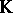. An arrangement of hyperplanes,, is a finite collection of codimension-one affine subspaces in, [a5].

### Examples of arrangements of hyperplanes.

1) A subset of the coordinate hyperplanes is called a Boolean arrangement.

2) An arrangement is in general position if at each point it is locally Boolean.

3) The braid arrangement consists of the hyperplanes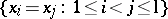. It is the set of reflecting hyperplanes of the symmetric group onletters.

4) The reflecting hyperplanes of a finite reflection group.

## Combinatorics.

An edgeofis a non-empty intersection of elements of. Let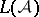be the set of edges partially ordered by reverse inclusion. Thenis a geometric semi-lattice with minimal element, rank given by codimension, and maximal elements of the same rank,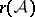. The Möbius function on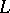is defined byand, for,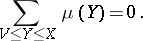The characteristic polynomial ofis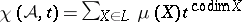. Let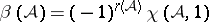. For a generic arrangement ofhyperplanes,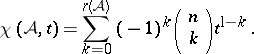For the braid arrangement,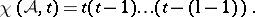Similar factorizations hold for all reflection arrangements involving the (co)exponents of the reflection group. Given a-tuple of hyperplanes,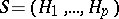, let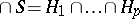; note thatmay be empty. One says thatis dependent if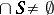and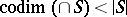. Let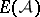be the exterior algebra on symbols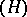for, where the product is juxtaposition. Define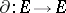by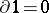,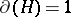and, for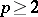,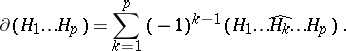Let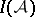be the ideal ofgenerated by. The Orlik–Solomon algebra ofis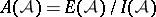. For connections with matroid theory, see [a3].

## Divisor.

The divisor ofis the union of the hyperplanes, denoted by. Ifor, thenhas the homotopy type of a wedge of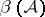spheres of dimension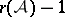, [a4]. The singularities of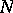are not isolated. The divisor of a general-position arrangement has normal crossings, but this is not true for arbitrary. Blowing-upalong all edges where it is not locally a product of arrangements yields a normal crossing divisor. See also Divisor.

## Complement.

The complement ofis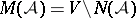.

1) If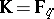, thenis a finite set of cardinality.

2) If, thenis a disjoint union of open convex sets (chambers) of cardinality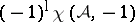. If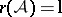,containschambers with compact closure, [a7].

3) If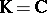, thenis an open complex (Stein) manifold of the homotopy type of a finite CW-complex (cf. also Stein manifold). Its cohomology is torsion-free and its Poincaré polynomial (cf. Künneth formula) is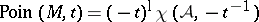. The product structure is determined by the isomorphism of graded algebras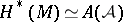. The fundamental group ofhas an effective presentation, but the higher homotopy groups ofare not known in general.

The complement of a Boolean arrangement is a complex torus. In a general-position arrangement of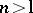hyperplanes,has non-trivial higher homotopy groups. For the braid arrangement,is called the pure braid space and its higher homotopy groups are trivial. The symmetric group acts freely onwith as orbit space the braid space whose fundamental group is the braid group. The quotient of the divisor by the symmetric group is called the discriminant, which has connections with singularity theory.

## Ball quotients.

Examples of algebraic surfaces whose universal cover is the complex ball were constructed as "Kummer" covers of the projective plane branched along certain arrangements of projective lines, [a2].

## Logarithmic forms.

For, choose a linear polynomialwith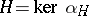and let. Let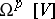denote all global regular (i.e., polynomial)-forms on. Letdenote the space of all global rational-forms on. The space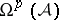of logarithmic-forms with poles alongis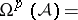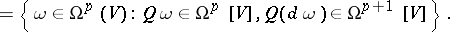The arrangement is free if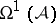is a free module over the polynomial ring. A free arrangementhas integer exponents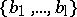, so that. Reflection arrangements are free. This explains the factorization of their characteristic polynomials.

## Hypergeometric integrals.

Certain rank-one local system cohomology groups ofmay be identified with spaces of hypergeometric integrals, [a1]. If the local system is suitably generic, these cohomology groups may be computed using the algebra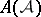. Only the top cohomology group is non-zero, and it has dimension. See [a6] for connections with the representation theory of Lie algebras and quantum groups, and with the Knizhnik–Zamolodchikov differential equations of physics.

How to Cite This Entry:
Arrangement of hyperplanes. Encyclopedia of Mathematics. URL: http://encyclopediaofmath.org/index.php?title=Arrangement_of_hyperplanes&oldid=24369
This article was adapted from an original article by P. Orlik (originator), which appeared in Encyclopedia of Mathematics - ISBN 1402006098. See original article